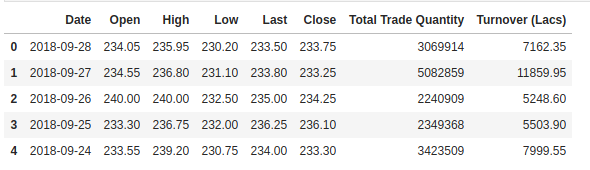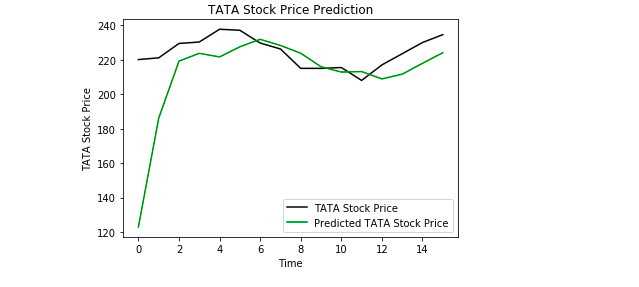>科技>正文

# 【DS】利用Keras长短期记忆(LSTM)模型预测股票价格

1 您如何处理时间序列数据？

LSTMs在序列预测问题中非常强大，因为它们能够存储过去的信息。这在我们的案例中很重要，因为股票的前一个价格对于预测其未来的价格是至关重要的。LSTMs在序列预测问题中非常强大，因为它们能够存储过去的信息。这在我们的案例中很重要，因为股票的前一个价格对于预测其未来的价格是至关重要的。

1importnumpy asnp

2importmatplotlib.pyplot asplt

3importpandas aspd

2training_set= dataset_train.iloc[:, 1: 2].values1from sklearn.preprocessing import MinMaxScaler

2sc = MinMaxScaler(feature_range = (0, 1))

3training_set_scaled = sc.fit_transform(training_set)

LSTMs期望我们的数据具有特定的格式，通常是一个3D数组。我们首先在60个时间步骤中创建数据，然后使用NumPy将其转换为数组。接下来，我们将数据转换为具有X_train示例、60个时间戳和每个步骤一个特征的3D维度数组。

1X_train = []

2y_train = []

3for i in range(60, 2035):

4X_train.append(training_set_scaled[i-60:i, 0])

5y_train.append(training_set_scaled[i, 0])

6X_train, y_train = np.array(X_train), np.array(y_train)

7

8X_train = np.reshape(X_train, (X_train.shape, X_train.shape, 1))

• Sequential用于初始化神经网络
• Dense用于添加密集连接的神经网络层
• LSTM用于添加长短期内存层
• Dropout用于添加防止过拟合的dropout层
1 fromkeras.models importSequential

2fromkeras.layers importDense

3fromkeras.layers importLSTM

4fromkeras.layers importDropout

• 50个单元，也就是输出空间的维度
• return_sequence =True，它决定是否返回输出序列中的最后一个输出，还是返回完整的序列
• input_shape作为训练集的shape

1regressor = Sequential()

2

3regressor. add(LSTM(units = 50, return_sequences = True, input_shape = (X_train.shape[ 1], 1)))

5

6regressor. add(LSTM(units = 50, return_sequences = True))

8

9regressor. add(LSTM(units = 50, return_sequences = True))

11

12regressor. add(LSTM(units = 50))

14

15regressor. add(Dense(units = 1))

16

17regressor.compile(optimizer = 'adam', loss = 'mean_squared_error')

18

19regressor.fit(X_train, y_train, epochs = 100, batch_size = 32)

2real_stock_price= dataset_test.iloc[:, 1: 2].values

1. 在0轴上合并训练集和测试集。
2. 将时间步长设置为60(如前所述)
3. 使用MinMaxScaler转换新数据集
4. 如前所述，重新塑造数据集

1dataset_total = pd.concat((dataset_train['Open'], dataset_test['Open']), axis = 0)

2inputs = dataset_total[len(dataset_total) - len(dataset_test) - 60:].values

3inputs = inputs.reshape(-1,1)

4inputs = sc.transform(inputs)

5X_test = []

6for i in range(60, 76):

7X_test.append(inputs[i-60:i, 0])

8X_test = np.array(X_test)

9X_test = np.reshape(X_test, (X_test.shape, X_test.shape, 1))

10predicted_stock_price = regressor.predict(X_test)

11predicted_stock_price = sc.inverse_transform(predicted_stock_price)

1plt.plot(real_stock_price, color = 'black', label= 'TATA Stock Price')

2plt.plot(predicted_stock_price, color = 'green', label= 'Predicted TATA Stock Price')

3plt.title( 'TATA Stock Price Prediction')

4plt.xlabel( 'Time')

5plt.ylabel( 'TATA Stock Price')

6plt.legend()

7plt.show()https://heartbeat.fritz.ai/using-a-keras-long-shortterm-memory-lstm-model-to-predict-stock-prices-a08c9f69aa74